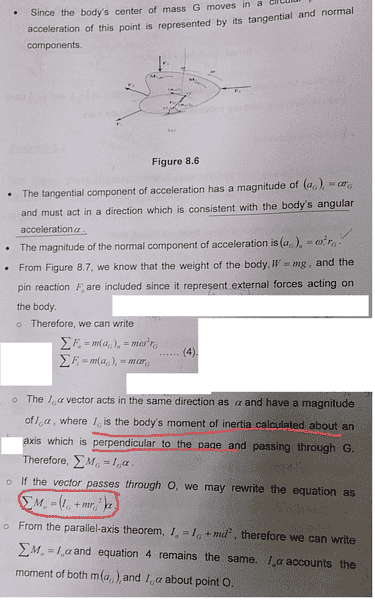in this notes , the author gave that the IG is the moment of inertia calculated about an axis which is perpendicular to the page .....

moment of inertia calculated about an axis which is perpendicular to the page here means id due to Ft(tangential force) only ? not include Fn ( normal force ) ?

So , for the sum of moment about O , it's involve only moment due to Ft only ? Not include Fn ? Why ? can you explain ?